# Defective value

(diff) ← Older revision | Latest revision (diff) | Newer revision → (diff)

of a meromorphic functionA complex number(finite or infinite) whose defect(see below) is positive. Let the functionbe defined in the disc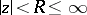of the complex plane. The defect (or deficiency) of the valueis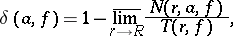where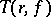is Nevanlinna's characteristic function representing the growth offor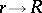, andis the counting function; here,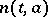is the number of solutions of the equationin(counted with multiplicity). If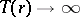as, thenfor all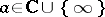. If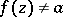for any, then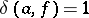andis a defective value; this equality also holds in some other cases (e.g.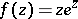,and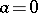).

If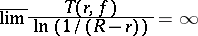(or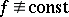is meromorphic throughout the plane), then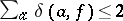(the defect, or deficiency, relation), and the number of defective values for suchis at most countable. Otherwise, the set of defective values may be arbitrary; thus, for any sequencesand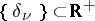,, it is possible to find an entire functionsuch that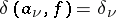for alland there are no other defective values of. Limitations imposed on the growth ofentail limitations on the defective values and their defects. For instance, a meromorphic function of order zero or an entire function of order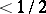cannot have more than one defective value.

The number(meromorphic in) is known as the defect in the sense of Valiron. The set of numbersfor which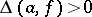may have the cardinality of the continuum, but always has logarithmic capacity zero.

How to Cite This Entry:
Defective value. Encyclopedia of Mathematics. URL: http://encyclopediaofmath.org/index.php?title=Defective_value&oldid=18447
This article was adapted from an original article by E.M. Chirka (originator), which appeared in Encyclopedia of Mathematics - ISBN 1402006098. See original article